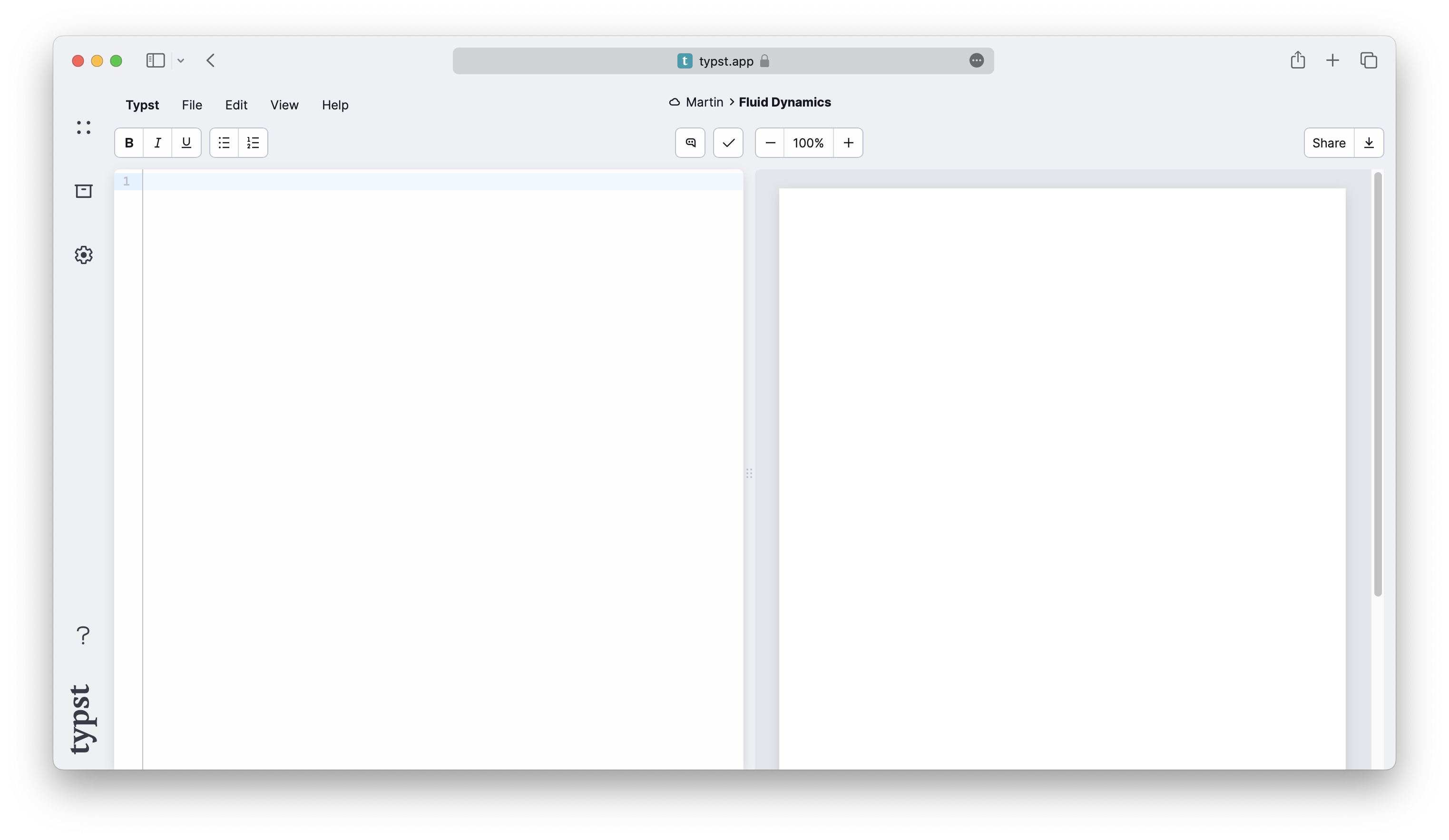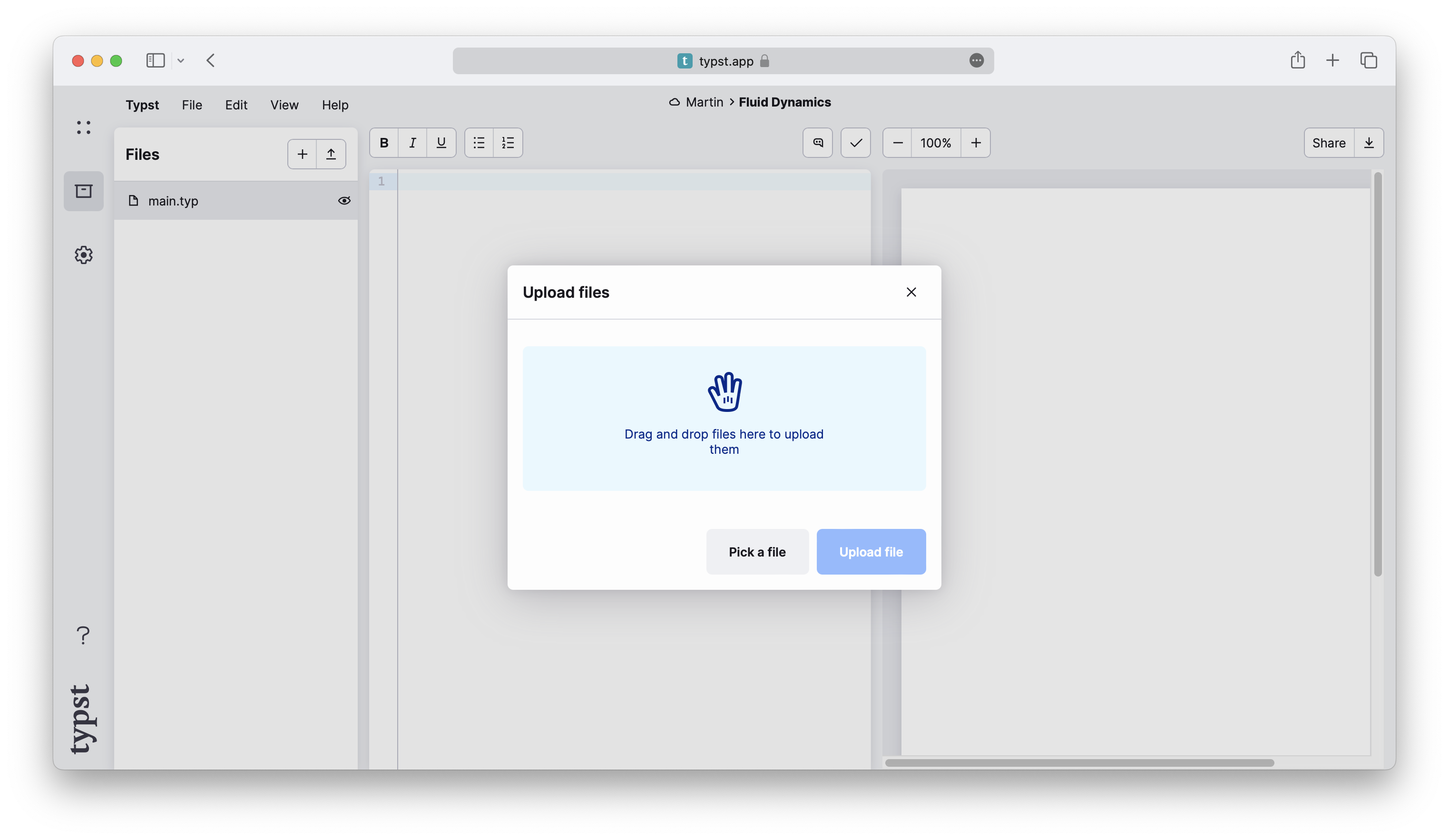Documentation

# Writing in Typst

Let's get started! Suppose you got assigned to write a technical report for university. It will contain prose, maths, headings, and figures. To get started, you create a new project on the Typst app. You'll be taken to the editor where you see two panels: A source panel where you compose your document and a preview panel where you see the rendered document.You already have a good angle for your report in mind. So let's start by writing the introduction. Enter some text in the editor panel. You'll notice that the text immediately appears on the previewed page.

``````In this report, we will explore the
various factors that influence fluid
dynamics in glaciers and how they
contribute to the formation and
behavior of these natural structures.
``````Throughout this tutorial, we'll show code examples like this one. Just like in the app, the first panel contains markup and the second panel shows a preview. We shrunk the page to fit the examples so you can see what's going on.

The next step is to add a heading and emphasize some text. Typst uses simple markup for the most common formatting tasks. To add a heading, enter the `=` character and to emphasize some text with italics, enclose it in `_underscores_`.

``````= Introduction
In this report, we will explore the
various factors that influence _fluid
dynamics_ in glaciers and how they
contribute to the formation and
behavior of these natural structures.
``````That was easy! To add a new paragraph, just add a blank line in between two lines of text. If that paragraph needs a subheading, produce it by typing `==` instead of `=`. The number of `=` characters determines the nesting level of the heading.

Now we want to list a few of the circumstances that influence glacier dynamics. To do that, we use a numbered list. For each item of the list, we type a `+` character at the beginning of the line. Typst will automatically number the items.

``````+ The climate
+ The topography
+ The geology
``````If we wanted to add a bulleted list, we would use the `-` character instead of the `+` character. We can also nest lists: For example, we can add a sub-list to the first item of the list above by indenting it.

``````+ The climate
- Temperature
- Precipitation
+ The topography
+ The geology
``````You think that your report would benefit from a figure. Let's add one. Typst supports images in the formats PNG, JPEG, GIF, and SVG. To add an image file to your project, first open the file panel by clicking the box icon in the left sidebar. Here, you can see a list of all files in your project. Currently, there is only one: The main Typst file you are writing in. To upload another file, click the button with the arrow in the top-right corner. This opens the upload dialog, in which you can pick files to upload from your computer. Select an image file for your report.We have seen before that specific symbols (called markup) have specific meaning in Typst. We can use `=`, `-`, `+`, and `_` to create headings, lists and emphasized text, respectively. However, having a special symbol for everything we want to insert into our document would soon become cryptic and unwieldy. For this reason, Typst reserves markup symbols only for the most common things. Everything else is inserted with functions. For our image to show up on the page, we use Typst's `image` function.

``````#image("glacier.jpg")
``````In general, a function produces some output for a set of arguments. When you call a function within markup, you provide the arguments and Typst inserts the result (the function's return value) into the document. In our case, the `image` function takes one argument: The path to the image file. To call a function, we first need to type the `#` character, immediately followed by the name of the function. Then, we enclose the arguments in parentheses. Typst recognizes many different data types within argument lists. Our file path is a short string of text, so we need to enclose it in double quotes.

The inserted image uses the whole width of the page. To change that, pass the `width` argument to the `image` function. This is a named argument and therefore specified as a `name: value` pair. If there are multiple arguments, they are separated by commas, so we first need to put a comma behind the path.

``````#image("glacier.jpg", width: 70%)
``````The `width` argument is a relative length. In our case, we specified a percentage, determining that the image shall take up `70%` of the page's width. We also could have specified an absolute value like `1cm` or `0.7in`.

Just like text, the image is aligned at the left side of the page by default. That looks a bit awkward. Let's center it and add a caption. We achieve this by using the `align` function. This function takes an `alignment` as its first argument and then the content that we want to align as the second argument:

``````#align(center)[
#image("glacier.jpg", width: 70%)

_Glaciers form an important
part of the earth's climate
system._
]
``````Did you spot the closing bracket `]` at the end of the example? Both the argument and the caption are enclosed in the same square brackets. Therefore, the `align` function centers both of them.

But wait, shouldn't the arguments of a function be specified within parentheses? Why is there a second set of square brackets with the aligned content after the parentheses?

The answer is that, as passing content to a function is such a common thing to do in Typst, there is special syntax for it: Instead of putting the content inside of the argument list, you can write it in square brackets directly after the normal arguments, saving on punctuation. We call markup in square brackets a content block.

So far, we've passed content blocks (markup in square brackets) and strings (text in double quotes) to our functions. Both seem to contain text. What's the difference?

A content block can contain text, but also any other kind of markup, function calls, and more, whereas a string is really just a sequence of characters and nothing else.

For example, the image function expects a path to an image file. It would not make sense to pass, e.g., a paragraph of text or a another image as the image's path parameter. That's why only strings are allowed here. On the contrary, strings work wherever content is expected because text is a kind of valid content.

## Maths

After fleshing out the introduction, you move on to the meat of the document: Your equations. Typst has built-in mathematical typesetting and uses its own math notation. Let's start with a simple equation. We wrap it in `\$` signs to let Typst know it should expect a mathematical expression:

``````The equation \$Q = rho A v + C\$
defines the glacial flow rate.
``````The equation is typeset inline, on the same line as the surrounding text. If you want to have it on its own line instead, you should insert a single space at its start and end:

``````The flow rate of a glacier is
defined by the following equation:

\$ Q = rho A v + C \$
``````We can see that Typst displayed the single letters `Q`, `A`, `v`, and `C` as-is, while it translated `rho` into a Greek letter. Math mode will always show single letters verbatim. Multiple letters, however, are interpreted as symbols, variables, or function names. To imply a multiplication between single letters, put spaces between them.

If you want to have a variable that consists of multiple letters, you can enclose it in quotes:

``````The flow rate of a glacier is given by
the following equation:

\$ Q = rho A v + "time offset" \$
``````You'll also need a sum formula in your paper. We can use the `sum` symbol and then specify the range of the summation in sub- and superscripts:

``````Total displaced soil by glacial flow:

\$ 7.32 beta +
sum_(i=0)^nabla Q_i / 2 \$
``````To add a subscript to a symbol or variable, type a `_` character and then the superscript. Similarly, use the `^` character for a superscript. If your sub- or superscript consists of multiple things, you must enclose them in round parentheses.

The above example also showed us how to insert fractions: Simply put a `/` character between the numerator and the denominator and Typst will automatically turn it into a fraction. Parentheses are smartly resolved, so you can enter your expression as you would into a calculator and Typst will replace parenthesized sub-expressions with the appropriate notation.

``````Total displaced soil by glacial flow:

\$ 7.32 beta +
sum_(i=0)^nabla
(Q_i (a_i - epsilon)) / 2 \$
``````Not all math constructs have special syntax. Instead, we use functions, just like the `image` function we have seen before. For example, to insert a column vector, we can use the `vec` function. Within math mode, function calls don't need to start with the `#` character.

``````\$ v := vec(x_1, x_2, x_3) \$
``````Some functions are only available within math mode. For example, the `cal` function is used to typeset calligraphic letters commonly used for sets. The math section of the reference provides a complete list of all functions that math mode makes available.

One more thing: Many symbols, such as the arrow, have a lot of variants. You can select among these variants by appending a dot and a modifier name to a symbol's name:

``````\$ a arrow.squiggly b \$
``````This notation is also available in markup mode, but the complete symbol name with modifiers must then be enclosed in colons. See the documentation of the text and math sections for more details.

## Review

You have now seen how to write a basic document in Typst. You learned how to emphasize text, write lists, insert images, align content, and typeset mathematical expressions. You also learned about Typst's functions. There are many more kinds of content that Typst lets you insert into your document, such as tables, shapes, and code blocks. You can peruse the reference to learn more about these and other features.

For the moment, you have completed writing your report. You have already saved a PDF by clicking on the download button in the top right corner. However, you think the report could look a bit less plain. In the next section, we'll learn how to customize the look of our document.